Home » Online Computer Education » Learn Fundamentals of Java Programming “Data Types and Variables in Java Programming ” Lesson 3

# Learn Fundamentals of Java Programming “Data Types and Variables in Java Programming ” Lesson 3

Data Types and Variables

In the previous section, you learnt how to write and execute a simple Java program. This time let us write a program that does something a little more useful than just display a message. We will write a program that handles data. Given the marks obtained by a student and the total number of marks for an exam, our program will calculate the percentage obtained by the student. And we will calculate the percentage for two students.

Variables

To store the program data we will use variables. A variable is a quantity whose value can change during program execution. Technically, a variable is the name for a storage location in the computer’s internal memory. The value of the variable is the contents at that location. In our percentage calculator program, we will use three variables named marks_obtained, the total_marks and percentage (Variable names have to be a single word, that’s why we have added an underscore between the words “marks” and “obtained”, “total” and “marks”).

All data variables in Java have to be declared and initialized before they are used. When declaring variables, we have to specify the data type of information that the member will hold – integer, fractional, alphanumeric, and so on. The type of a variable tells the compiler how much memory to reserve when storing a variable of that type.

In our percentage calculator program, the variable total_marks can have only integer values. So we declare it to be of type int – a keyword in Java that indicates an integer datatype. The variables obtained_marks, percentage are declared of type

double, since they can have fractional values (floating point numbers). Inside the main method, we declare these variables, we also assign them values using the = operator as shown below:

int total_marks = 400 ;
double marks_obtained = 346;
double percentage = 0.0 ;

To calculate the percentage, we construct an expression using the total_marks and marsk_obtained variables and assign the value to the percentage variable. The * operator is used for multiplication and the / operator for division.

percentage = (marks_obtained/total_m arks)*100 ;

To display the percentage in the IDE output window, we use our old friend – the System.out.prinltn() method.

System.out.println(“Student1 ‘s Percentage = “+percentage);

Notice that the variable to be displayed is not put within the double quotes. To print the word Percentage, we put it inside double quotes, to display the value stored in the percentage variable, we put it outside the double quotes. The + operator within the System.out.println statement, concatenates the string given in double quotes and the value of the variable.
To calculate the percentage for another student, we reuse the variables. We change the value of the variable marks_obtained and calculate the percentage again. There is no need to change the total_marks because they remain the same as before.

marks_obtained = 144 ;
percentage = (marks_obtained/total_marks)*100 ;
System.out.println(“Student2 ‘s Percentage = “+percentage);

To write the percentage calculator program in NetBeans, follow the steps given below:
Step 1: First, we will create a new Class file within the package helloworld (that was created in the previous section). Click on File > New File (Ctrl + N) to create a new file (Figure 1.2a).Step 2: In the New File dialog box that appears, under the Categories list, select Java and under the File Types list select Java Class (they should be already selected). Click on Next to create a new Java Class file (Figure 1.2b).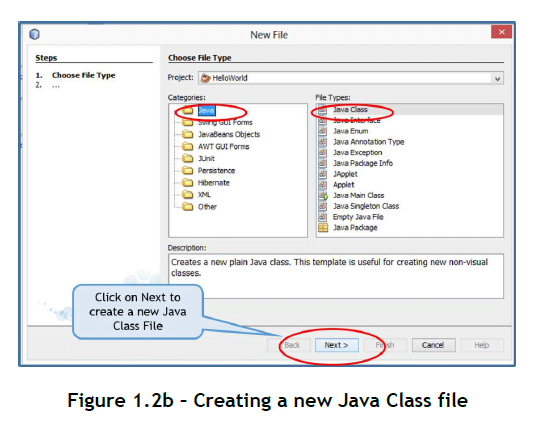Step 3: In the New Java Class dialog box that appears, type a name in the Class Name textbox (Figure 1.2c). For our program, we type PercentageCalculator. When you type the name of the class, the Created File field also changes automatically. Make sure the Package field displays the helloworld package, if not click on the drop down list icon next to the field and select the helloworld package. Click on Finish to finish creating the New Java Class File and to return back to the IDE.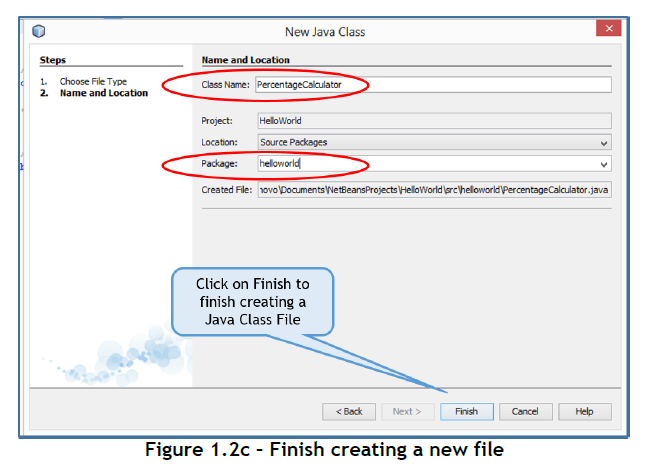Observe that in the Projects tab, a file called PercentageCalculator.java has been added to the helloworld package (Figure 1.2d).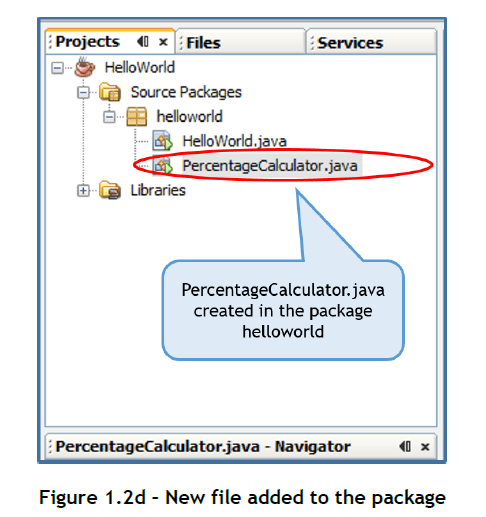Step 4: Click in the Code Editor Window under the Percentage Calculator.java tab and type the code as shown in Figure 1.2e. The comments inserted by NetBeans have been deleted for simplicity.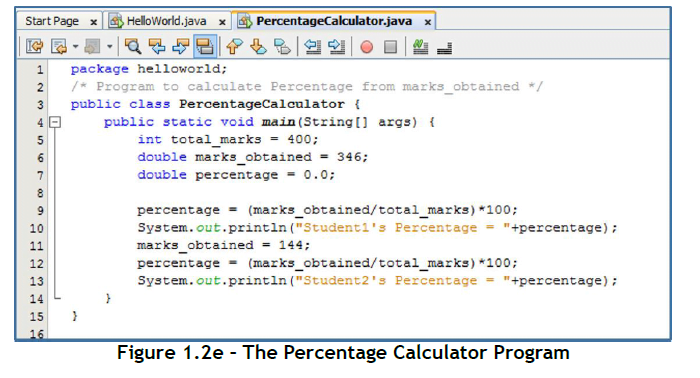Step 5: Click File > Save (Ctrl+S) to save the file.
Step 6: Click Run > Run File (Shift + F6) to execute the program. The output of the program appears in the Output window as shown in Figure 1.2f.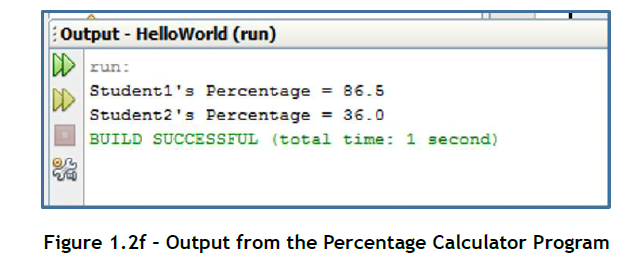As you may have noticed, the variables in a program act as placeholders for data handled by the program. Variables can change their value during program execution. The variables that you have seen in this section are local variables. Such variables are available only inside the methods where they are declared.The main objective of this website is to provide quality study material to all students (from 1st to 12th class of any board) irrespective of their background as our motto is “Education for Everyone”. It is also a very good platform for teachers who want to share their valuable knowledge.